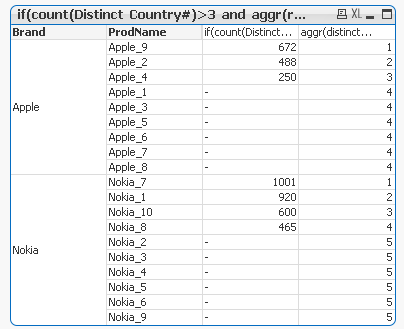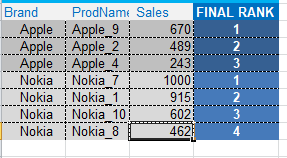# QlikView App Dev

Discussion Board for collaboration related to QlikView App Development.

Announcements
cancel
Showing results for
Did you mean:Champion III

## Fixate Rank ( IF

Hi Guys,

I want to be able to fixate the rank ignoring all selections in ProdName for Brand Field

Currently I have the below table, but the rank changes on selections in the Prodname field even after adding {1} to all aggregationsCurrent Expression:

=AGGR(rank(sum({1}AGGR(

if(count(distinct Country#)>3      //sale rows in more than 3 countries

and

AGGR(rank(sum({1}Sales),4,1),Brand,ProdName)<=5  // Ranks among Top 5 within the Brand

, sum({1}Sales)),

Brand,ProdName)),4,1),Brand,ProdName)

Expected Output:Vineeth Pujari
If a post helps to resolve your issue, please accept it as a Solution.
1 Solution

Accepted SolutionsMVP

Or use this expressions

IF(Rank(SUM({1}Sales),4,1)<=5,

Aggr(Rank(SUM({1<ProdName = {"=COUNT({1}DISTINCT Country#)>3"}>}Sales),4,1)

,Brand,ProdName))

and

Aggr(

IF(Rank(SUM({1}Sales),4,1)<=5,

SUM({1<ProdName = {"=COUNT({1}DISTINCT Country#)>3"}>}Sales))

,Brand,ProdName)

2 RepliesMVP

I am not able to get the exact output you have mentioned...

Rank is wrong and Sales is also wrong..

Try my below suggestion and let me know if you think this not work for you..

Create a Pivot Table

Dimension

Brand

ProdName

Expression

Aggr(

IF(Rank(SUM({1<ProdName = {"=COUNT({1}DISTINCT Country#)>3"}>}Sales),4,1)<=5,

SUM({1}Sales))

,Brand,ProdName)

and

Aggr(

IF(Rank(SUM({1<ProdName = {"=COUNT({1}DISTINCT Country#)>3"}>}Sales),4,1)<=5,

Rank(SUM({1<ProdName = {"=COUNT({1}DISTINCT Country#)>3"}>}Sales),4,1))

,Brand,ProdName)MVP

Or use this expressions

IF(Rank(SUM({1}Sales),4,1)<=5,

Aggr(Rank(SUM({1<ProdName = {"=COUNT({1}DISTINCT Country#)>3"}>}Sales),4,1)

,Brand,ProdName))

and

Aggr(

IF(Rank(SUM({1}Sales),4,1)<=5,

SUM({1<ProdName = {"=COUNT({1}DISTINCT Country#)>3"}>}Sales))

,Brand,ProdName)